# 【论文阅读】Fully Convolutional Networks for Semantic Segmentation

## FCN，shift-and-stitch，backwards convolution（deconvolution）

Posted by x-jeff on January 31, 2022

# 1.Introduction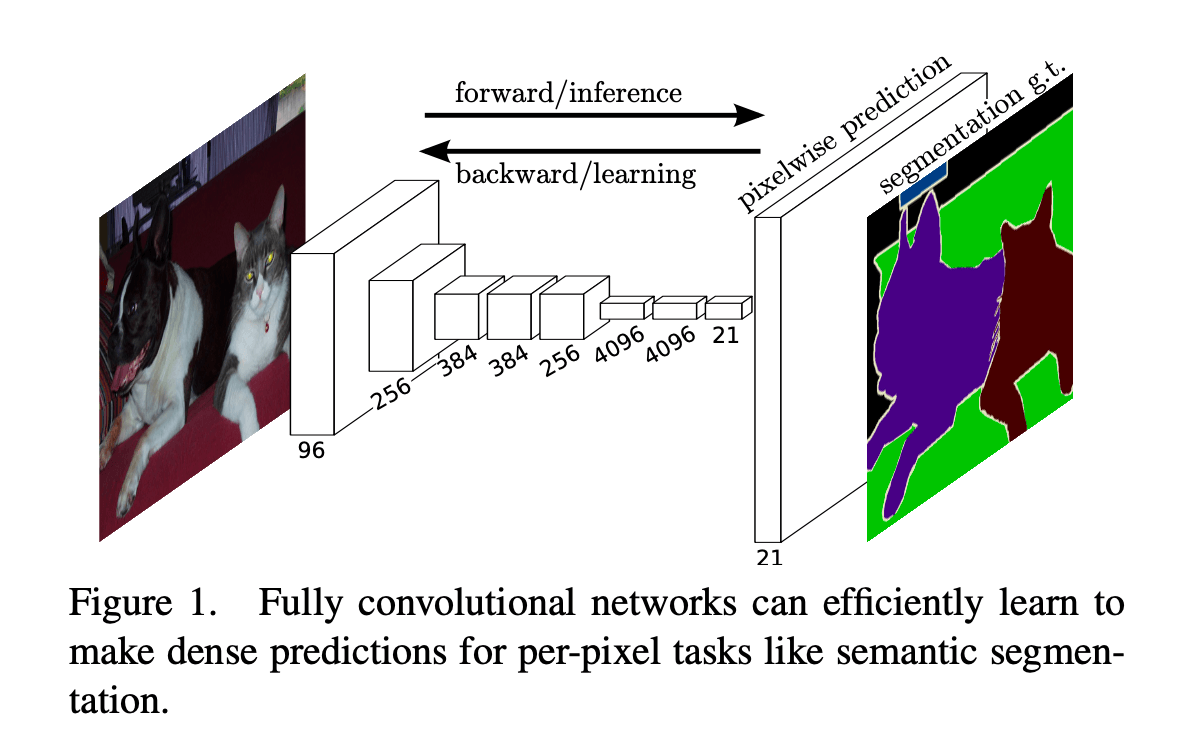dense prediction：预测出图像中每个像素点的所属类别。

pixelwise：像素级别。
patchwise：patch级别。
imagewise：图像级别。

# 2.Related work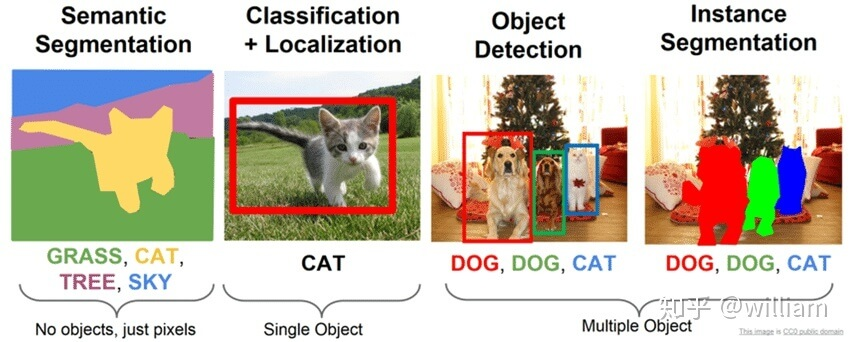👉Fully convolutional networks

👉Dense prediction with convnets

• capacity和receptive fields受限的小型模型。
• patchwise级别的训练。
• 都采用了一些后处理的方法，例如superpixel projection, random field regularization, filtering或者local classification。
• 输入移位和dense输出的隔行交错输出。
• 多尺度金字塔处理。
• 饱和双曲正切非线性。
• 集成。

NYUDv2（NYU Depth Dataset V2）数据集由微软Kinect的RGB和Depth摄像机记录的各种室内场景的视频序列组成。它的特点：

1. 1449张标注的RGB图片和深度图。
2. 来自3个城市，464个场景。
3. 407024张没有标注的图片。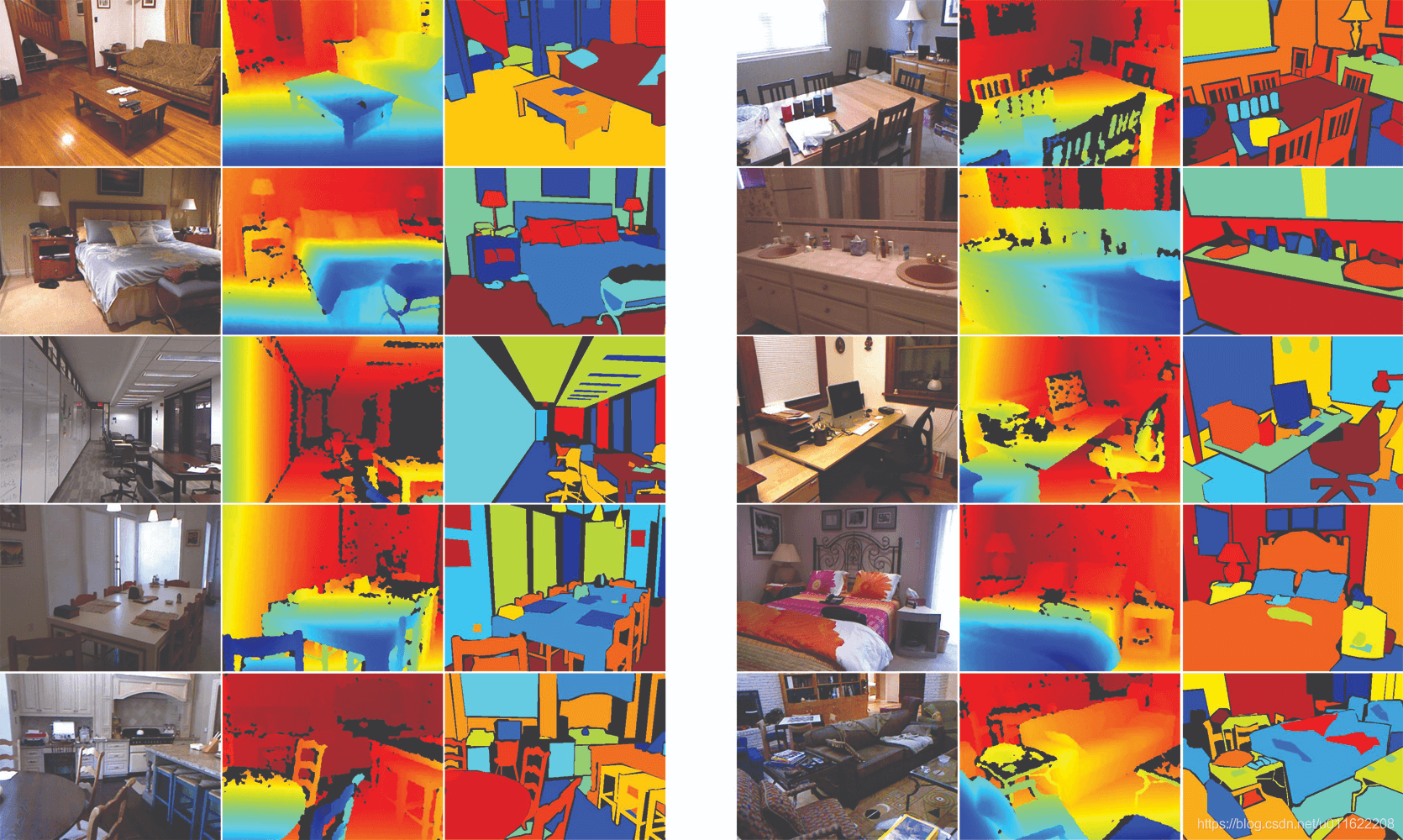# 3.Fully convolutional networks

$\mathbf{y}_{ij}=f_{ks} ( \{ \mathbf{x}_{si+\delta i , sj+\delta j} \} _ {0 \leqslant \delta i , \delta j \leqslant k})$

kernel的大小和步长遵循以下转换规则：

$f_{ks} \circ g_{k's'} = (f \circ g) _{k' + (k-1)s',ss'}$

$f \circ g$表示$f$与$g$的合成函数或复合函数。例如：$(g\circ f) (x) = g(f(x))$。

FCN使用实值损失函数（a real-valued loss function）。损失函数可定义为最后一层空间维度的和：

$\ell (\mathbf{x};\theta) = \sum_{ij} \ell ' (\mathbf{x}_{ij};\theta)$

## 3.1.Adapting classifiers for dense prediction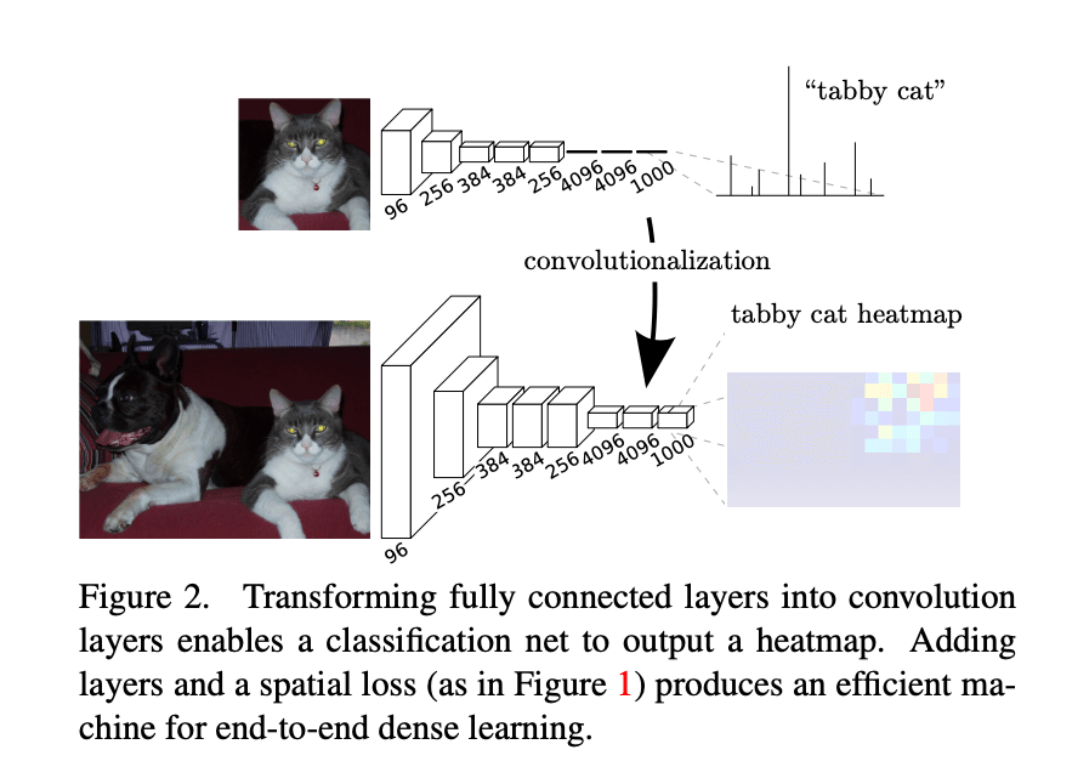Fig2展示了如何将全连接层转换成卷积层使其可以输出heatmap。添加层和spatial loss（如Fig1所示）可以为端到端的dense learning提供有效的机制。

grid中的每个点在输入层面的感受野都是$227 \times 227$，所以FCN相当于用了22ms预测了$10\times 10=100$幅$227 \times 227$patch的类别，而AlexNet预测100幅$227 \times 227$图像需要$1.2\times 100=120$ms，所以说快了将近5倍。

## 3.2.Shift-and-stitch is filter rarefaction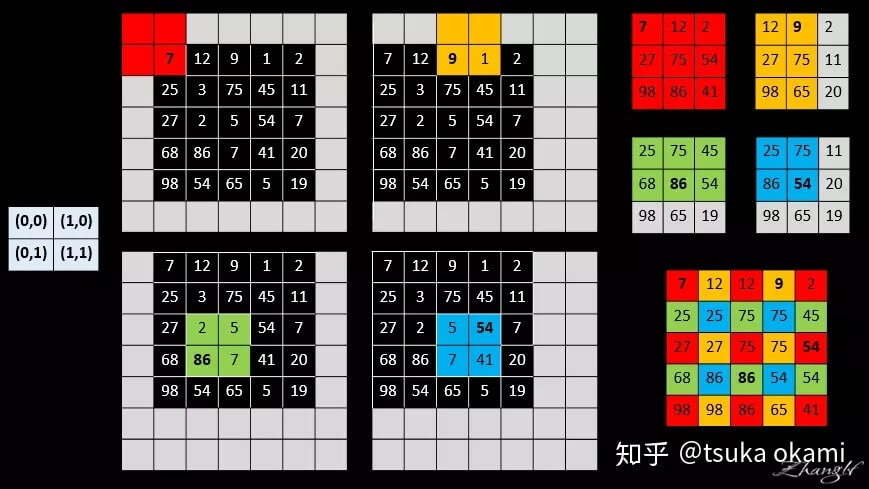shift-and-stitch这个方法会使计算成本增加$f^2$倍，但是有一个trick（即filter rarefaction）可以更有效的得到一样的结果。还是用上面的例子，如果把max pooling的stride设为1可以得到一样的结果。这个原理也很好理解，因为每次移动input一个像素就相当于input不动，移动filter一步。但是上述例子是假设网络只有一个max pooling层，而实际情况是网络通常由多个卷积层、pooling层组成，如果还是仅仅把下采样层的stride改为1无法得到相同的结果。解决这一问题的办法是扩展原来的filter。考虑某一下采样层，其stride=s，后接一个卷积层，卷积核权重为$f_{ij}$，记input大小为$w\times h$，想得到dense prediction有两种方式：

1. shift and stitch。过程为：shift input，得到$s^2$个inputs，然后分别经过subsampling layer得到$s^2$个outputs，这些outputs又分别通过后续的conv层，得到$s^2$个outputs，最后stitch这$s^2$个outputs得到最终大小为$w\times h$的output。
2. filter rarefaction。将下采样层的stride改为1，同时将后接卷积层的filter按如下方式dilate：

$f'_{ij} = \begin{cases} f_{i/s,j/s}, & \text{if s divides both i and j;} \\ 0, & \text{otherwise,} \end{cases}$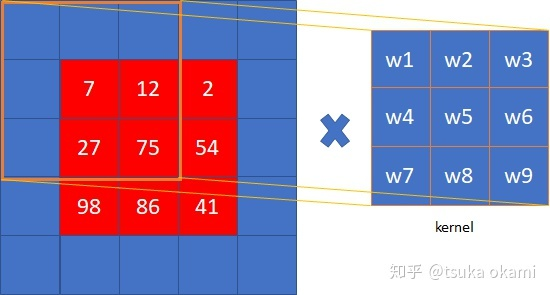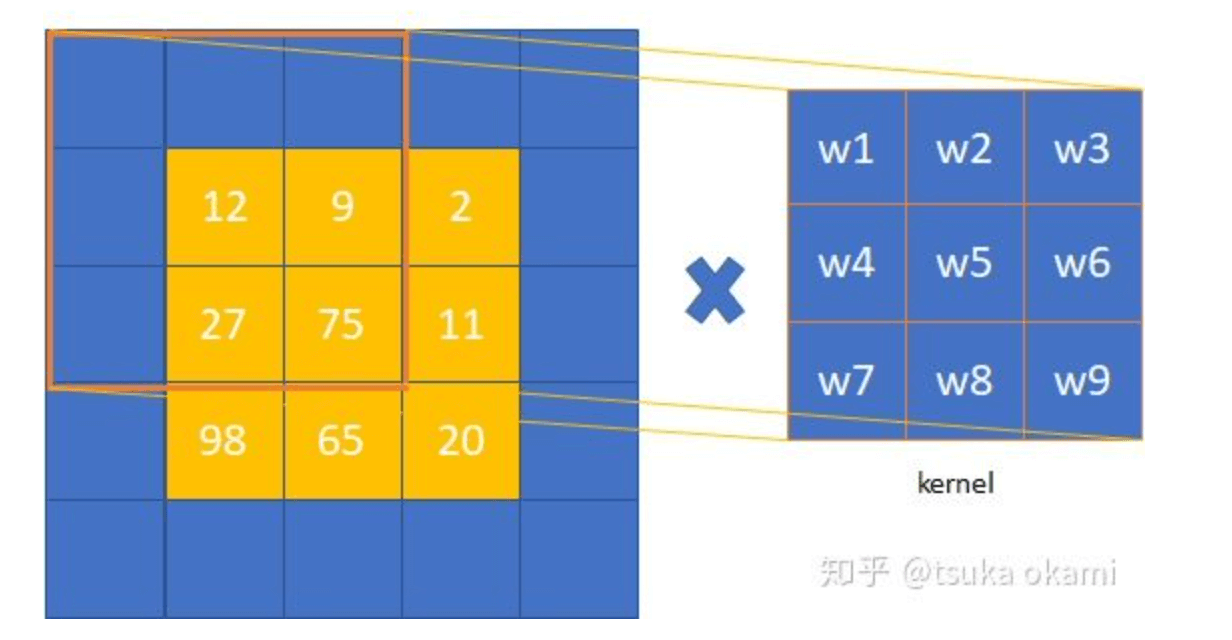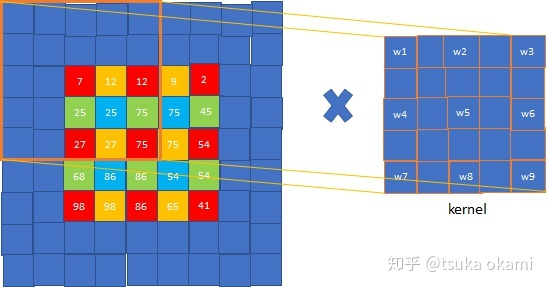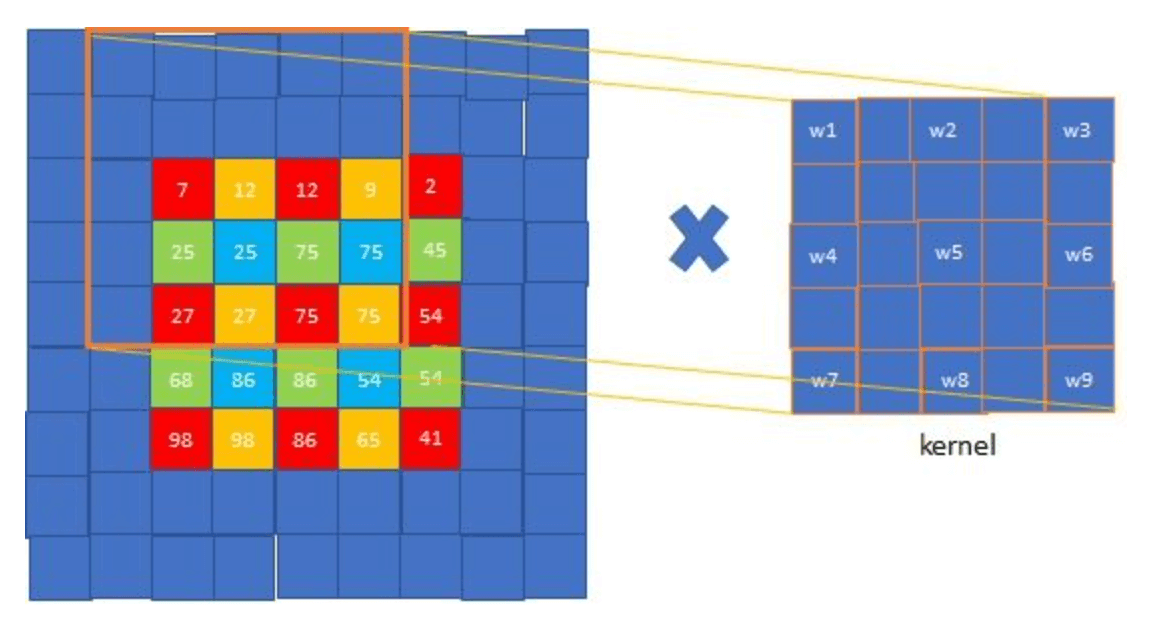## 3.3.Upsampling is backwards strided convolution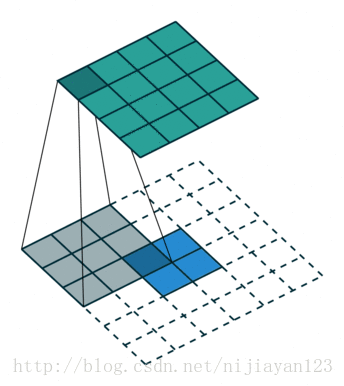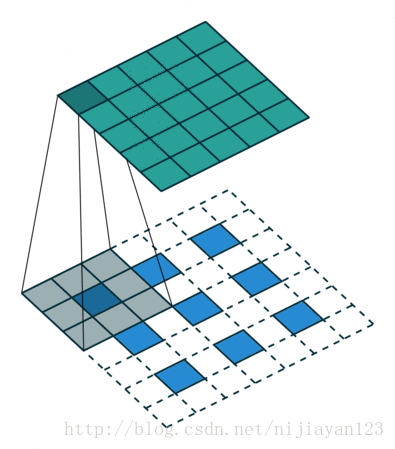## 3.4.Patchwise training is loss sampling

patchwise的训练和全卷积训练都可以产生任意的分布，其计算效率取决于重叠部分和minibatch的大小。

# 4.Segmentation Architecture

## 4.1.From classifier to dense FCN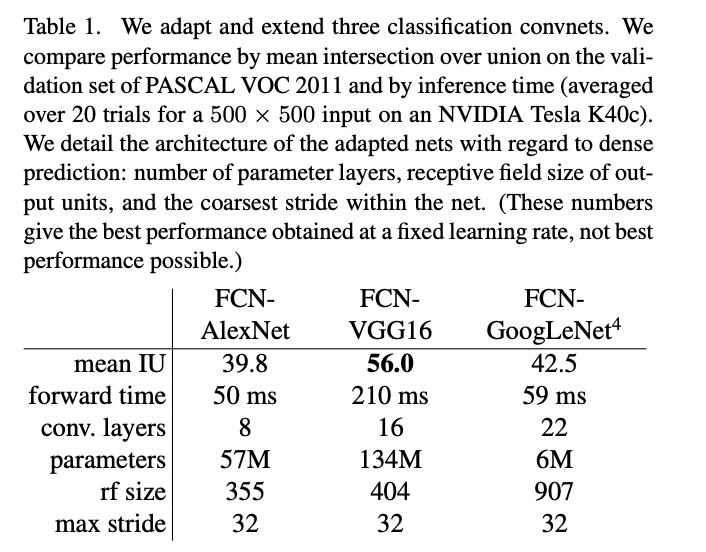## 4.2.Combining what and where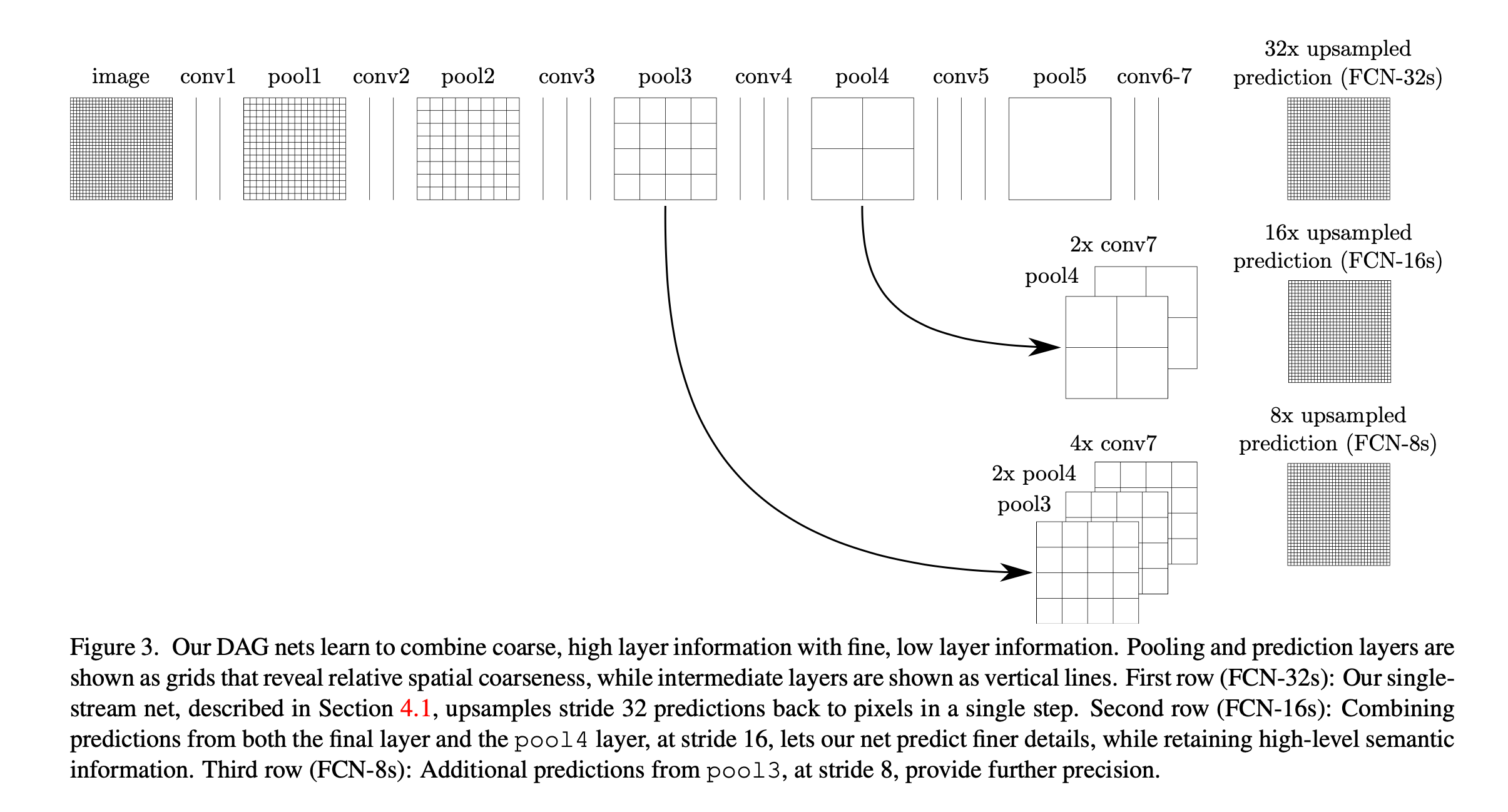Fig3也很好理解，第一行为普通的FCN结构，通过32倍上采样（即5次上采样），称为FCN-32s（第4.1部分的模型基本都属于FCN-32s）。为了能让浅层精细的信息和深层粗糙的信息结合起来，作者添加了skip。Fig3的第二行将conv7上采样一次（即2x）后和pool4相结合（可以理解为拼接在一起，二者维度是一样的），然后上采样4次（即16x），这种结构称为FCN-16s。类似的，Fig3的第三行，conv7上采样2次+pool4上采样1次+pool3拼接在一起，然后上采样3次（即8x），这样的结构称为FCN-8s。3种结构的结果见Fig4：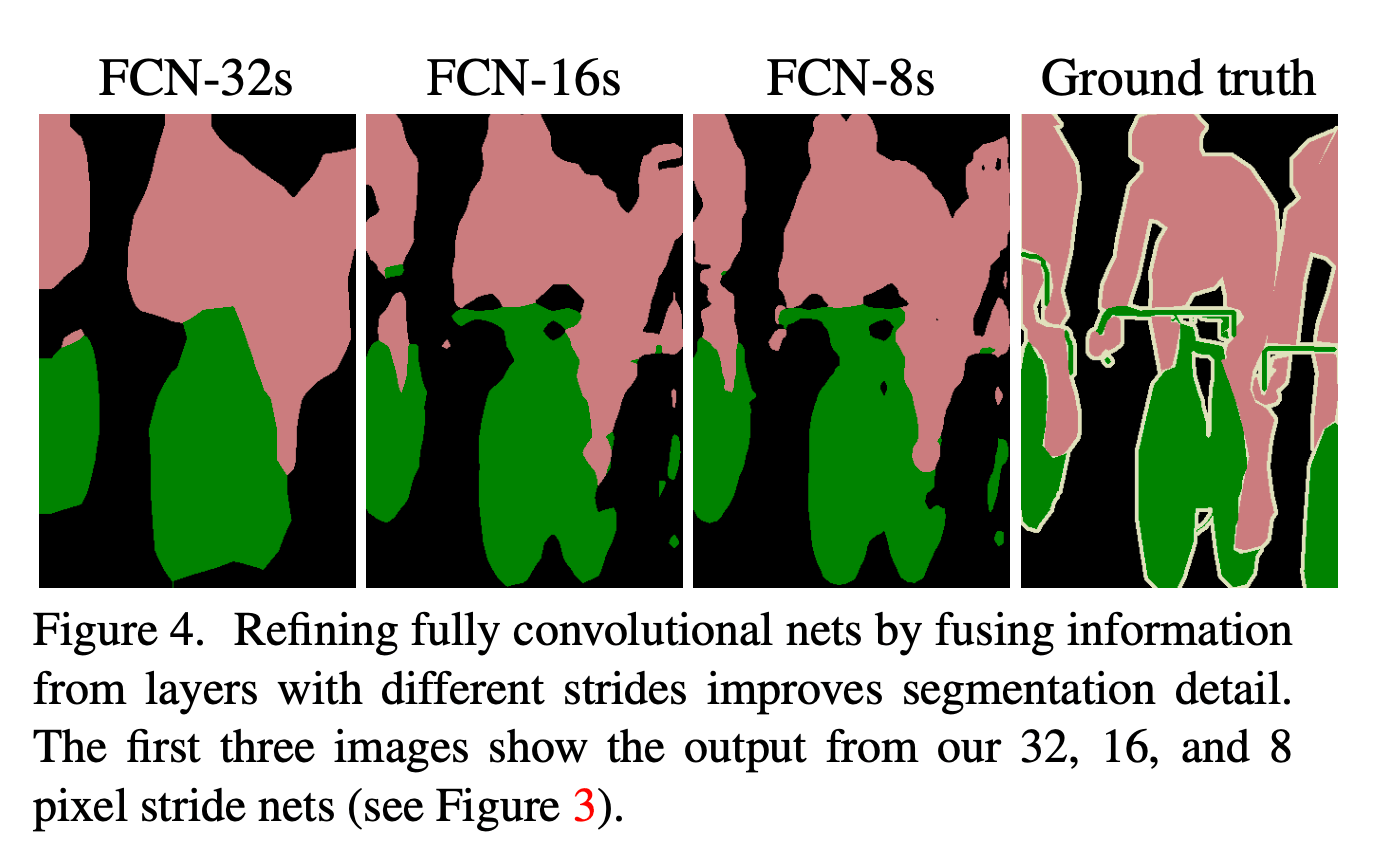FCN-32s的分割效果最差。FCN-16s稍微好一点，FCN-8s效果最优。

skip结构的加入将FCN-16s在验证集上的mean IU提升至62.4，FCN-8s在验证集上的mean IU提升至62.7。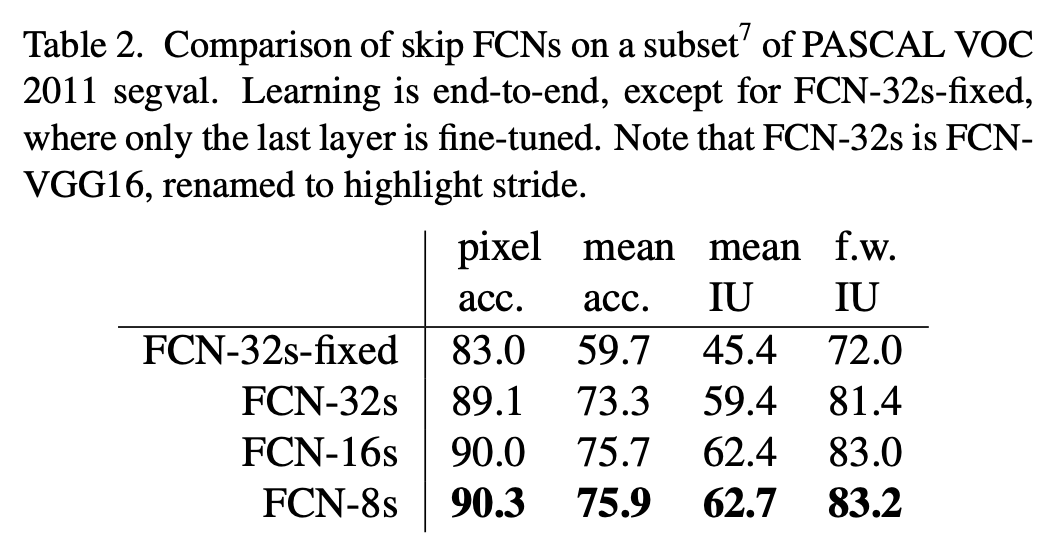👉Refinement by other means

## 4.3.Experimental framework

👉Optimization：训练使用MBGD+momentum，minibatch size=20。学习率是固定的，FCN-AlexNet的学习率为$10^{-3}$，FCN-VGG16的学习率为$10^{-4}$，FCN-GoogLeNet的学习率为$5^{-5}$。momentum=0.9，weight decay=$5^{-4}$或$2^{-4}$。我们使用0初始化class scoring layer，因为随机初始化既不能加快收敛也不能获得更好的性能。原始分类网络的dropout被保留。

👉Fine-tuning：我们fine-tune了所有层。从表2可以看到，只fine-tune最后一层只能获得fine-tune所有层70%的性能。考虑到训练原始分类网络所需要的时间，training from scratch显然是不可行的。在单块GPU上，通过fine-tune得到FCN-32s花了三天时间。然后又各花了一天时间升级得到FCN-16s和FCN-8s。

👉More Training Data：PASCAL VOC 2011分割训练集有1112张带标签图像。此外，又使用了“B. Hariharan, P. Arbelaez, L. Bourdev, S. Maji, and J. Malik. Semantic contours from inverse detectors. In International Conference on Computer Vision (ICCV), 2011.”提供的8498张带标签的PASCAL训练图像。更多的训练数据使得FCN-VGG16的mean IU提升了3.4，达到了59.4。

👉Patch Sampling：如Fig5所示，全图训练和patch sampling的效果差不多（见Fig5左），但是全图训练的收敛速度更快（见Fig5右）。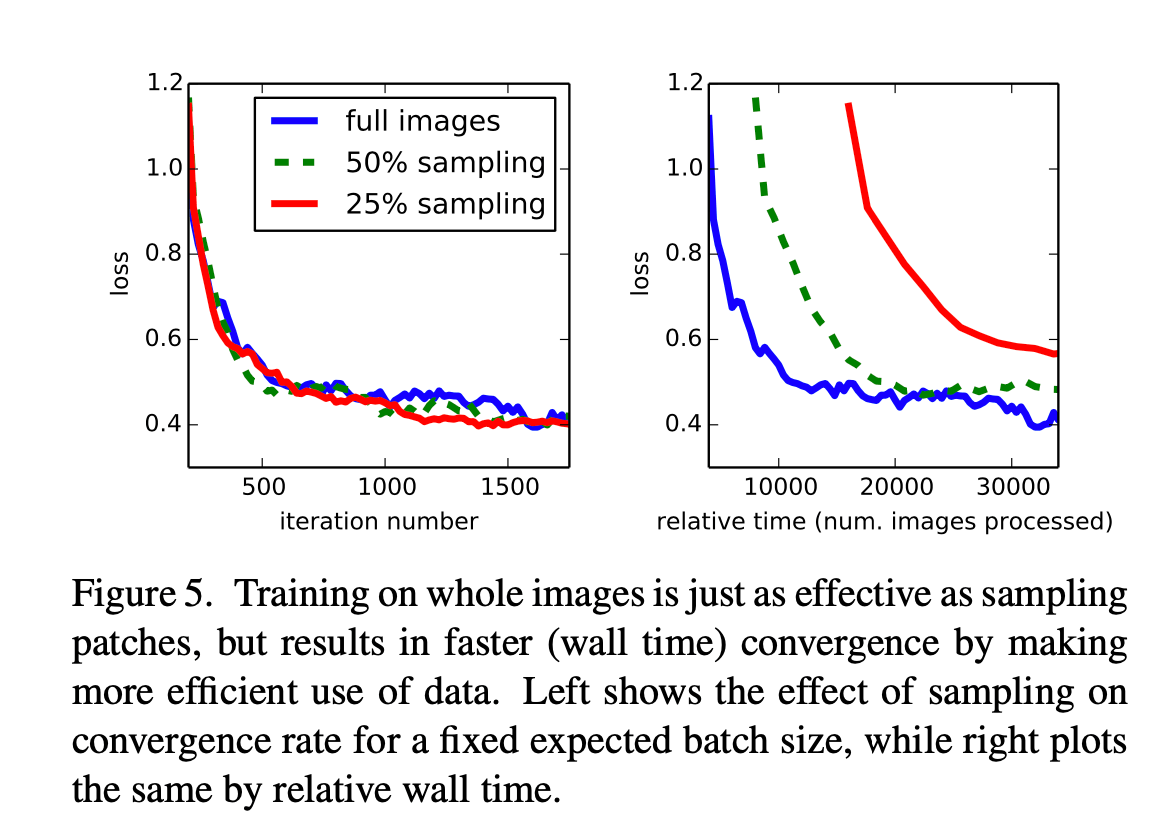👉Class Balancing：全卷积训练可以通过加权或采样的方法来进行类别平衡。虽然我们的类别很不平衡（大约3/4都是背景），但是我们发现类别平衡不是必要的。

👉Dense Prediction：通过反卷积得到和输入维度一样的输出。

👉Augmentation：我们尝试通过镜像和抖动来扩展训练数据集，但是发现并没有带来性能的提升。

👉Implementation：所有模型的训练和测试都使用Caffe，并基于单个NVIDIA Tesla K40c GPU。我们的模型以及代码都是开源的，地址：https://github.com/shelhamer/fcn.berkeleyvision.org

# 5.Results

👉Metrics：模型评估使用以下常用指标（$n_{ij}$表示类别$i$被预测为类别$j$的像素点个数，$t_i = \sum_j n_{ij}$表示真实类别为$i$的像素点的个数，$n_{cl}$表示类别个数）：

• pixel accuracy：

$\sum_{i} n_{ii} / \sum_i t_i$
• mean accuraccy：

$(1/n_{cl}) \sum_i n_{ii} / t_i$
• mean IU：

$(1/n_{cl}) \sum_i n_{ii} / (t_i +\sum_j n_{ji} - n_{ii})$
• frequency weighted IU：

$(\sum_k t_k)^{-1} \sum_i t_i n_{ii} / (t_i +\sum_j n_{ji} - n_{ii})$

👉PASCAL VOC：FCN-8s在PASCAL VOC 2011和2012测试集上的表现见表3。并和之前SOTA的方法：SDS、R-CNN进行比较。FCN-8s取得了最高的mean IU，并且推理速度也快了很多。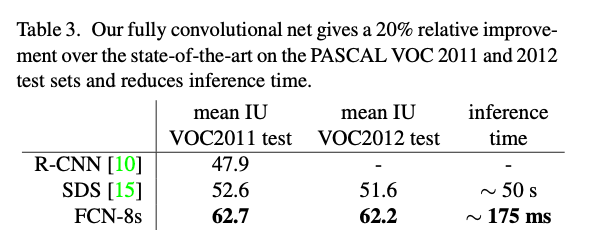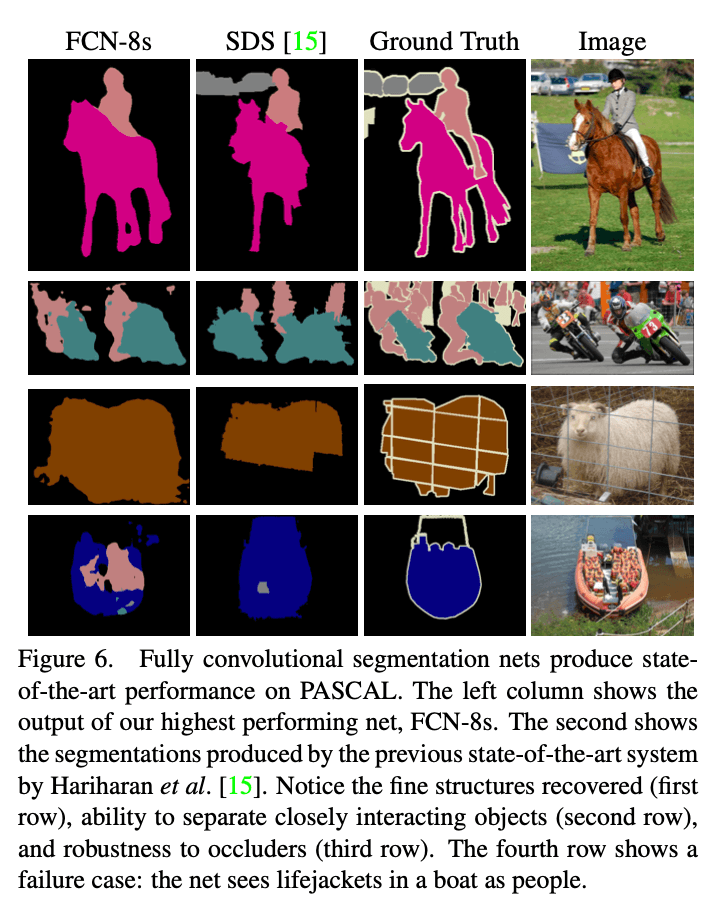👉NYUDv2：我们的测试结果基于标准的数据集：795张训练图像和654张测试图像。测试结果见表4。加入深度信息后，结果稍微变好了一点（FCN-32s RGB vs. FCN-32s RGBD）。我们也尝试了以HHA作为输入。最终，FCN-16s RGB-HHA取得了最好的结果。

HHA：

• H：horizontal disparity，水平视差。
• H：height above ground，离地高度。
• A：the angle of the local surface normal with the inferred gravity direction，局部表面法线与推断重力方向的角度。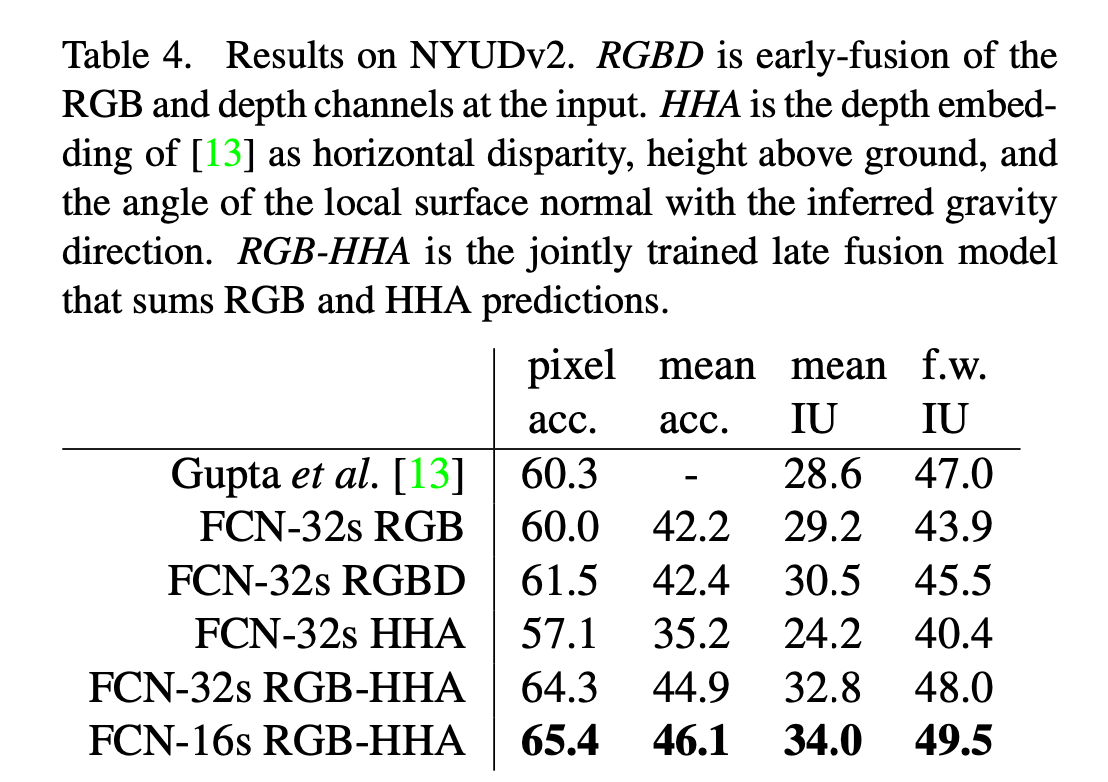👉SIFT Flow：该数据集包含有2688张带像素标签的图像，共有33个语义类别（例如桥、山、太阳等）和3个几何类别（水平、垂直和天空）。FCN可以学习并预测两种类型标签的联合表示。结果见表5。数据集划分为2488张训练图像和200张测试图像。FCN-16s在两种标签任务中都是表现最优的。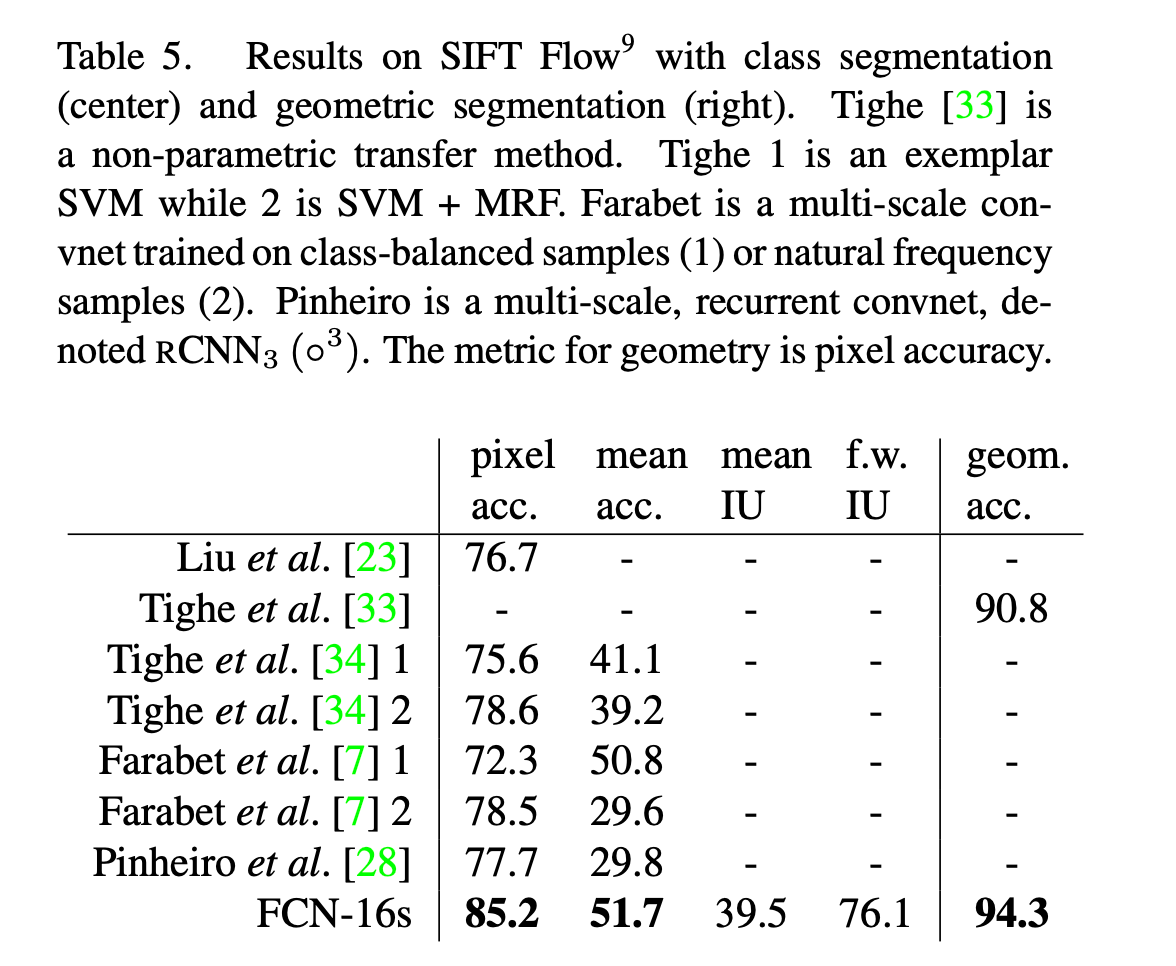# 6.Conclusion

FCN是一类丰富的模型，分类网络就是其中的一个特例。将分类网络扩展到语义分割，并通过multi-resolution layer combinations来改善网络结构，达到SOTA，并且简化和加快了学习和推理速度。

# 8.参考资料